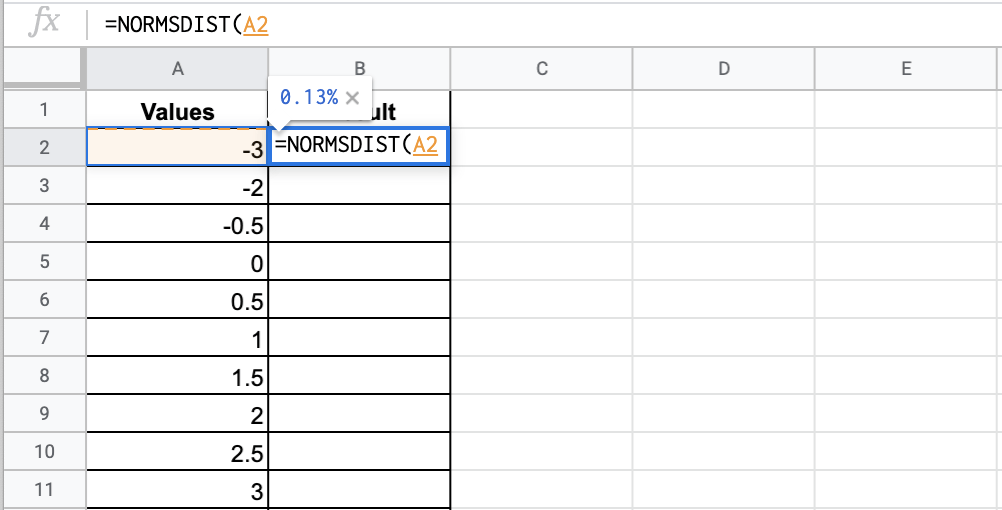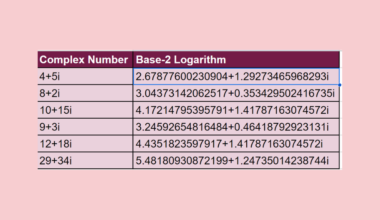# How to Use the NORMSDIST Function in Google Sheets

The `NORMSDIST` function in Google Sheets is useful for finding out the value of the standard normal distribution function given a specific value.

Google Sheets is an ideal tool for basic statistical functions. Laying out your data in the spreadsheet, there are various functions you can use to study the data and extract good insights from this survey.

Let’s take an example.

Suppose you are doing a statistical analysis and you want to find the probability that your normally distributed variable in the standard normal distribution is less than or equal to a value z.

In particular, it focuses on the standard normal cumulative distribution function, with the mean of zero and a standard deviation of 1. So it essentially functions like the `NORMDIST` function, except the mean and standard deviation will be set.

These values are used so often that there are tables made for these kinds of values. However, if you don’t have this table on hand, there is a certain function that should help you.

Thankfully, the `NORMSDIST` function can help us out with these questions.

We will discuss an example and actual values to help you understand the NORMSDIST function in Google Sheets.

## The Anatomy of the NORMSDIST Function

The syntax (the way we write) the `NORMSDIST` function is simple:

`=NORMSDIST(x)`

Let’s break this down to understand the syntax of the `NORMSDIST` function and each term:

• = the equal sign is how we begin any function in Google Sheets.
• `NORMSDIST` is our function. Make sure to type in NORMSDIST and forego the similar but different NORMDIST function.
• X is the input to the standard normal distribution function. Usually, this is the number that you are concerned with.

Note that the “standard” normal distribution function is the normal distribution function, which has a mean of 0 and a standard deviation of 1.

## A Real Example of Using the NORMSDIST Function

Let’s look at this example below to see how to use the `NORMSDIST` function in Google Sheets.In this simple problem, we want to find out the percentage of the curve is less that or equal to -3.

The function with a cell reference is:

`=NORSMDIST(A2)`

As a result, we get 0.13%.

This simple problem can be practiced. Use the link below to use our spreadsheet sample:

## How to Use the NORMSDIST function in Google Sheets

In this section, we will show you a step-by-step process on how to use the `NORMSDIST` function in Google Sheets.

### Finding the standard normal distribution

1. To begin, lay out your data accordingly in Google Sheets. Click on the cell where you want to show your result to make it an active cell.2. Next, type the equal sign ‘=’ to start off the function which is ‘NORMSDIST’.3. You can now see the auto-suggest box will pop up with the name of various functions. Make sure that you select the correct one.4. After entering an opening bracket, you will see more instructions on using the function.5. Next, you will need to add the x input. You can directly enter the text or use a cell reference, like in the example.6. Hit enter or return, and a closing bracket will be automatically added for you. Your answer should be displayed in the result cell.That’s all, good job! You can now use the `NORMSDIST` function together with other numerous Google Sheets formulas to create effective and powerful spreadsheets!Our goal this year is to create lots of rich, bite-sized tutorials for Google Sheets users like you. If you liked this one, you'll love what we are working on! Readers receive ✨ early access ✨ to new content. There will be no spam and you can unsubscribe at any time.

##### You May Also Like## How to Use the IMABS Function in Google Sheets

The IMABS function in Google Sheets returns the absolute value (the modulus) of a complex number. A complex…## How to Count Rows Between Two Values in Google Sheets

Knowing how to count rows between two values in Google Sheets is useful if you want to find…## How to Find the Last Value in Each Row in Google Sheets

To find the last value in each row in Google Sheet using the LOOKUP function is useful if…## How to Use IMLOG2 Function in Google Sheets

This guide will explain how to use the IMLOG2 function in Google Sheets. When we need to calculate…